Covariance Designs

# Covariance Designs

## Design Notation

The basic Analysis of Covariance Design (ANCOVA or ANACOVA) is a just pretest-posttest randomized experimental design. The notation shown here suggests that the pre-program measure is the same one as the post-program measure (otherwise we would use subscripts to distinguish the two), and so we would call this a pretest. But you should note that the pre-program measure doesn’t have to be a pretest – it can be any variable measured prior to the program intervention. It is also possible for a study to have more than one covariate.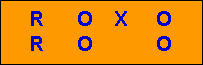The pre-program measure or pretest is sometimes also called a “covariate” because of the way it’s used in the data analysis – we “covary” it with the outcome variable or posttest in order to remove variability or noise. Thus, the ANCOVA design falls in the class of a “noise reduction” experimental design (see Classifying the Experimental Designs).

In social research we frequently hear about statistical “adjustments” that attempt to control for important factors in our study. For instance, we might read that an analysis “examined posttest performance after adjusting for the income and educational level of the participants.” In this case, “income” and “education level” are covariates. Covariates are the variables you “adjust for” in your study. Sometimes the language that will be used is that of “removing the effects” of one variable from another. For instance, we might read that an analysis “examined posttest performance after removing the effect of income and educational level of the participants.”

## How Does A Covariate Reduce Noise?

Let’s begin with data from a simple ANCOVA design as described above. The first figure shows the pre-post bivariate distribution. Each “dot” on the graph represents the pretest and posttest score for an individual. We use an ‘X’ to signify a program or treated case and an ‘O’ to describe a control or comparison case. You should be able to see a few things immediately. First, you should be able to see a whopping treatment effect! It’s so obvious that you don’t even need statistical analysis to tell you whether there’s an effect (although you may want to use statistics to estimate its size and probability). How do I know there’s an effect? Look at any pretest value (value on the horizontal axis). Now, look up from that value – you are looking up the posttest scale from lower to higher posttest scores. Do you see any pattern with respect to the groups? It should be obvious to you that the program cases (the ‘X’s) tend to score higher on the posttest at any given pretest value. Second, you should see that the posttest variability has a range of about 70 points.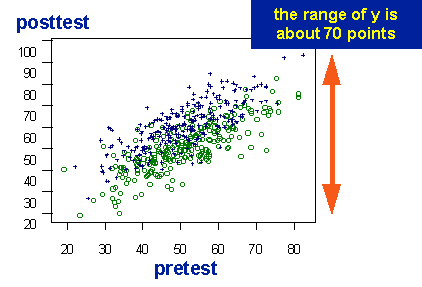Now, let’s fit some straight lines to the data. The lines on the graph are regression lines that describe the pre-post relationship for each of the groups. The regression line shows the expected posttest score for any pretest score. The treatment effect is even clearer with the regression lines. You should see that the line for the treated group is about 10 points higher than the line for the comparison group at any pretest value.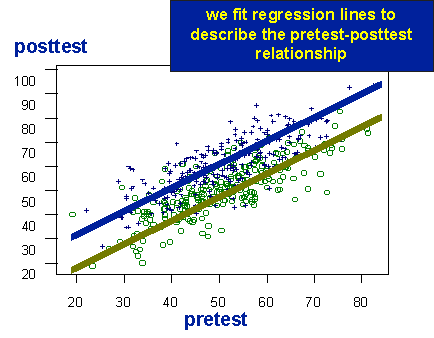What we want to do is remove some of the variability in the posttest while preserving the difference between the groups. Or, in other terms, we want to “adjust” the posttest scores for pretest variability. In effect, we want to “subtract out” the pretest. You might think of this as subtracting the line from each group from the data for each group. How do we do that? Well, why don’t we actually subtract?!? Find the posttest difference between the line for a group and each actual value. We call each of these differences a residual – it’s what’s left over when you subtract a line from the data.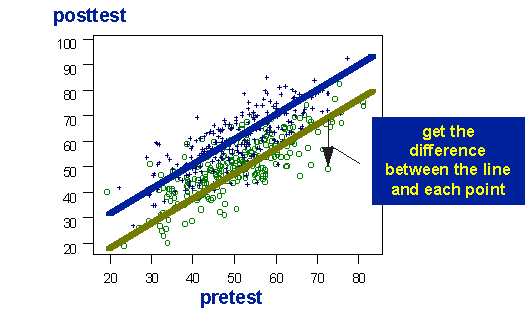Now, here comes the tricky part. What does the data look like when we subtract out a line? You might think of it almost like turning the above graph clockwise until the regression lines are horizontal. The figures below show this in two steps. First, I construct and x-y axis system where the x dimension is parallel to the regression lines.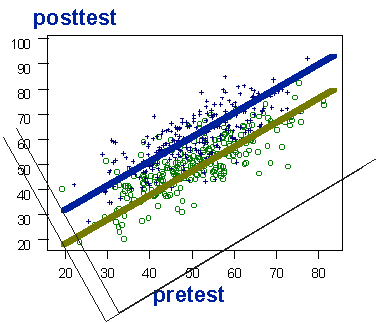Then, I actually turn the graph clockwise so that the regression lines are now flat horizontally. Now, look at how big the posttest variability or range is in the figure (as indicated by the red double arrow). You should see that the range is considerably smaller that the 70 points we started out with above. You should also see that the difference between the lines is the same as it was before. So, we have in effect reduced posttest variability while maintaining the group difference. We’ve lowered the noise while keeping the signal at its original strength. The statistical adjustment procedure will result in a more efficient and more powerful estimate of the treatment effect.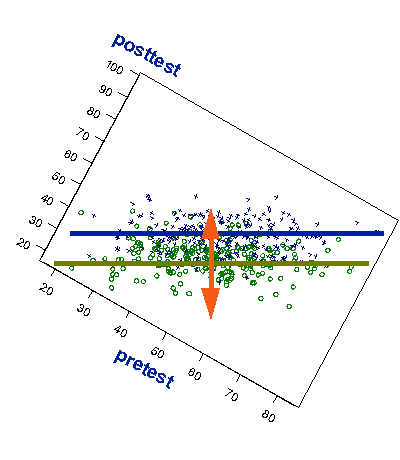You should also note the shape of the pre-post relationship. Essentially, the plot now looks like a zero correlation between the pretest and, in fact, it is. How do I know it’s a zero correlation? Because any line that can be fitted through the data well would be horizontal. There’s no slope or relationship. And, there shouldn’t be. This graph shows the pre-post relationship after we’ve removed the pretest! If we’ve removed the pretest from the posttest there will be no pre-post correlation left.

Finally, let’s redraw the axes to indicate that the pretest has been removed. here, the posttest values are the original posttest values minus the line (the predicted posttest values). That’s why we see that the new posttest axis has 0 at it’s center. Negative values on the posttest indicate that the original point fell below the regression line on the original axis. Here, we can better estimate that the posttest range is about 50 points instead of the original 70, even though the difference between the regression lines is the same. We’ve lowered the noise while retaining the signal.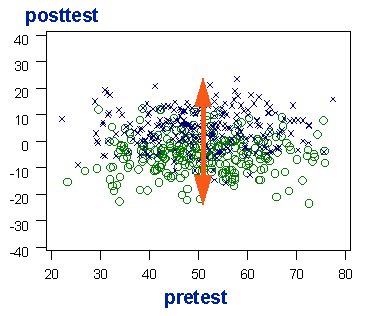[DISCLAIMER: OK, I know there’s some statistical hot-shot out there fuming about the inaccuracy in my description above. My picture rotation is not exactly what we do when we adjust for a covariate. My description suggests that we drop perpendicular lines from the regression line to each point to obtain the subtracted difference. In fact, we drop lines that are perpendicular to the horizontal axis, not the regression line itself (in Least Squares regression we are minimizing the the sum of squares of the residuals on the dependent variable, not jointly on the independent and dependent variable). In any event, while my explanation may not be perfectly accurate from a statistical point of view, it’s not very far off, and I think it conveys more clearly the idea of subtracting out a relationship. I thought I’d just put this disclaimer in to let you know I’m not dumb enough to believe that the description above is perfectly accurate.]

The adjustment for a covariate in the ANCOVA design is accomplished with the statistical analysis, not through rotation of graphs. See the Statistical Analysis of the Analysis of Covariance Design for details.

## Summary

Some thoughts to conclude this topic. The ANCOVA design is a noise-reducing experimental design. It “adjusts” posttest scores for variability on the covariate (pretest). This is what we mean by “adjusting” for the effects of one variable on another in social research. You can use any continuous variable as a covariate, but the pretest is usually best. Why? Because the pretest is usually the variable that would be most highly correlated with the posttest (a variable should correlate highly with itself, shouldn’t it?). Because it’s so highly correlated, when you “subtract it out” or “remove’ it, you’re removing more extraneous variability from the posttest. The rule in selecting covariates is to select the measure(s) that correlate most highly with the outcome and, for multiple covariates, have little intercorrelation (otherwise, you’re just adding in redundant covariates and you will actually lose precision by doing that). For example, you probably wouldn’t want to use both gross and net income as two covariates in the same analysis because they are highly related and therefore redundant as adjustment variables.